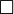Home > T-SQL > SQL SERVER – SQUARE() – Math Functions @SQLSERVER

## SQL SERVER – SQUARE() – Math Functions @SQLSERVER

SQUARE () function returns the square of a float expression given in the function argument. The return type of this function is float.is a geometry symbol to denotes square.

Syntax

SQUARE ( float expression )

Example

–Square a positive number
select square (3)
Output: 9

–Square a negative number
select square (-3)
Output: 9

–Square a decimal number
select square (0.25)
Output: 0.0625

Square of a value x can be represented  as (x * x). So, we can also use this method in our program. Built-in functions are useful to developers to build application easier and more readable.